# Iterate until a value is true before running a tool and use the Iteration value as a parameter for a tool

284
9
01-18-2022 07:45 AM
Labels (3)by
New Contributor III

Hi all,

I have a challenge I cant find a solution on in ArcGIS. This is so much easier for me in FME. But perhaps there is a elegant fix.

What I want: I want to devide polygons in sub areas. But the sub areas can not be less then 140000.
What I think I need to do:  To iterate Area/N with Area/N+1 until Area is 140000 or less. I will try to illustrate in the image below.

Ive tried to look at the iterations and logic functions but cant find any solution with them. So what I think I have to do is  perhaps to pre run  the field calculator tool  and add condition values with (N values( wich a can later use as a  parameter for the Subdivide tool.. But i am not sure. Am quite stuck.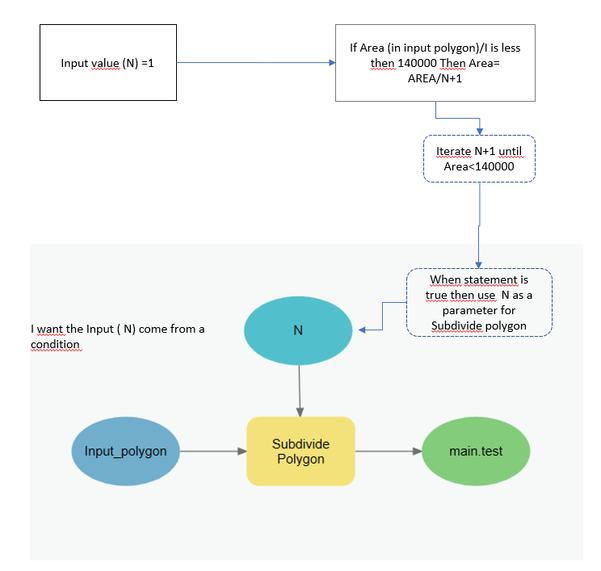Tags (5)
1 Solution

Accepted Solutionsby
New Contributor III

solved. Used Get Field Value instead. Output is Double so it can be calculated in the calculate value expression.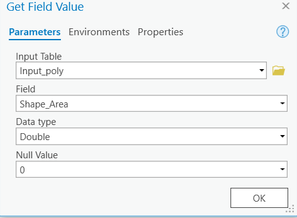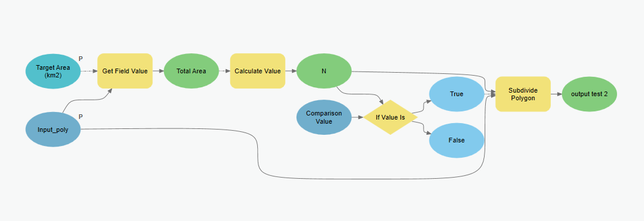9 RepliesbyMVP Esteemed Contributor

Which options would you be using from the subdivide tool?

There are lots of parameters to specify and your model doesn't indicate which ones you will be using

Subdivide Polygon (Data Management)—ArcGIS Pro | Documentation

... sort of retired...by
New Contributor III

"sort of retired"  Is there at better choice?byMVP Esteemed Contributor

That is my signature... and something others can aspire to ;)

... sort of retired...byMVP Esteemed Contributor

This tool has an option for equal areas, if you just provide 14000 it sounds like will give you what you want - no need for an iterator.by
New Contributor III

Long day,,, So true. OMG.

I need a brake. To much multitasking.byMVP Esteemed Contributor

No worries. Just for the good of the thread, I will mention that if you want know how many polygons you got at the end, you can run the Row Count tool on the output of Subdivide Polygon.by
New Contributor III

Thank you. But I think ive got new questions for my thread. In these example I use another fictive number.

One parameter that I did not tell was that my Input areas is going to be folder iterated since there are several areas.

Lets say I use Equal Areas where the target area is 4 and use the subdevision type= strips. That gives me a rest over. The the rest over can be very small. Lets say My Total Area is 8.4 in the first example. It will give me a rest  area on 0.4.  If I use stacked the areas will be 4.2 which I cannot use. So the area should be equal divided but less then a given sqm.

So If I stick to my first idea it give me a calculated result  8.2/x=4 Then X = 3.2 or  3 (INT) That gives me a result where all the areas are equal.

I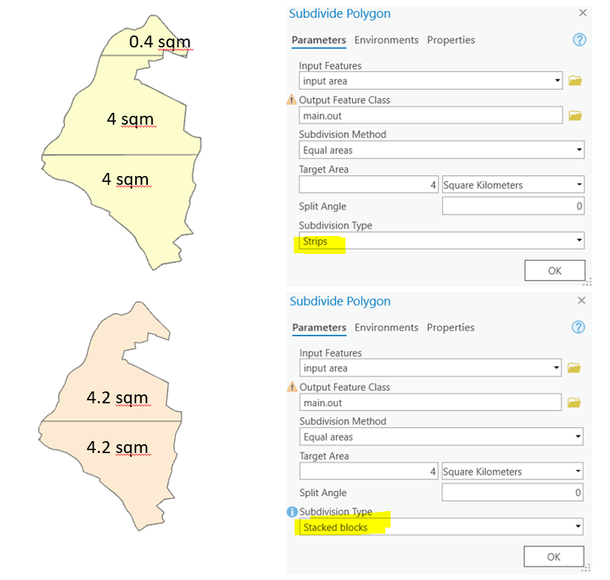by
New Contributor III

Ok, I am soon in goal.  Still not there yet

This is the model now:
Problem: I dont know if I get the correct values from Iterate field values. This tool get the Area value. But i dont know how to check if the output is the actual area.

It works like this. The Calculate value ( written by a collegue not me) need 2 input parameters. Target Area( entered by the user, and Total Area ( calculated from the Iterate field value ).
But the data "Total Area" dont give me the correct value to use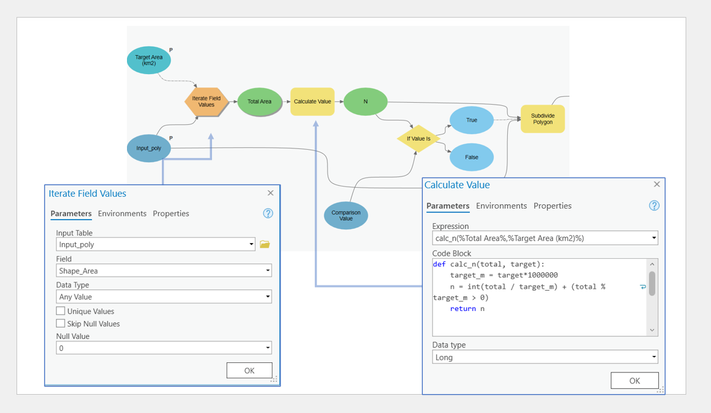by
New Contributor III

solved. Used Get Field Value instead. Output is Double so it can be calculated in the calculate value expression.